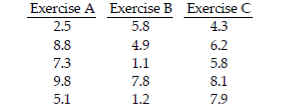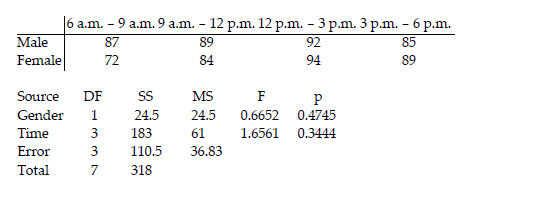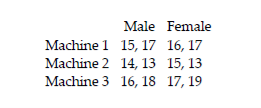• FAQ
• Contact/ Homework Answers / Statistics / Test the claim that the samples come from populations with
Not my Question
Flag Content

# Question

Test the claim that the samples come from populations with the same mean. Assume that the populations are normally distributed with the same variance.

12) The data below represent the weight losses for people on three different exercise programs.At the 1% significance level, does it appear that a difference exists in the true mean weight loss produced by the three exercise programs?

Use the data in the given table and the corresponding Minitab display to test the hypothesis.

13) The following table entries are test scores for males and females at different times of day. Assuming no effect from the interaction between gender and test time, test the claim that time of day does not affect test scores. Use a 0.05 significance level.Provide an appropriate response.

14) The following data contains task completion times, in minutes, categorized according to the gender of the machine operator and the machine used.Assume that two-way ANOVA is used to analyze the data. How are the ANOVA results affected if 5 minutes is added to each completion time?

15) Suppose you are to test for equality of four different population means, with H0: μA = μB = μC = μD. Write the hypotheses for the paired tests. Use methods of probability to explain why the process of ANOVA has a higher degree of confidence than testing each of the pairs separately.

16) Draw an example of an F distribution and list the characteristics of the F distribution.

## Solution 5 (1 Ratings )

Solved
Statistics 1 Month Ago 5 Views